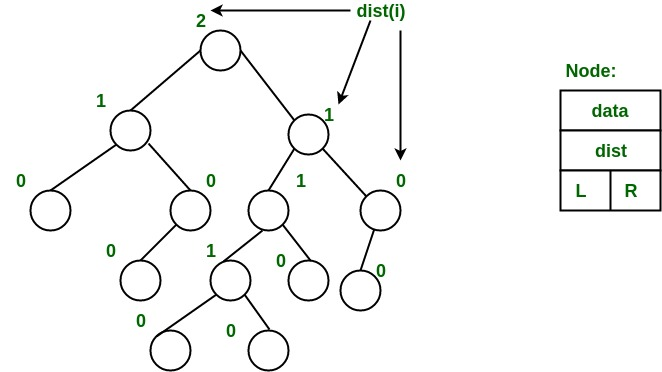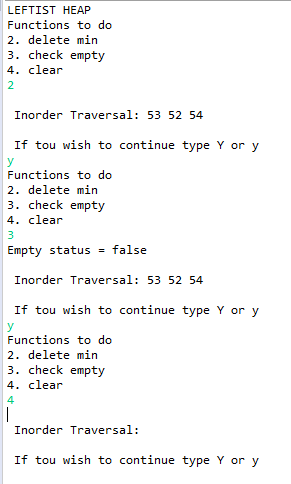# Java Program to Implement Leftist Heap

• Difficulty Level : Expert
• Last Updated : 13 Sep, 2021

A leftist heap is a priority Queue implemented with a binary heap. Every node has a sValue which is at the nearest Distance to the other nodes. Now we will write a java program for performing certain operations on a leftist Heap (Inorder Traversal) like insert, delete, clear, and check for empty.

A leftist tree is a binary tree with properties:

Attention reader! Don’t stop learning now. Get hold of all the important DSA concepts with the DSA Self Paced Course at a student-friendly price and become industry ready.  To complete your preparation from learning a language to DS Algo and many more,  please refer Complete Interview Preparation Course.

In case you wish to attend live classes with experts, please refer DSA Live Classes for Working Professionals and Competitive Programming Live for Students.

1. Normal Min Heap Property : key(i) >= key(parent(i))
2. Heavier on left side : dist(right(i)) <= dist(left(i)). Here, dist(i) is the number of edges on the shortest path from node i to a leaf node in extended binary tree representation (In this representation, a null child is considered as an external or leaf node). The shortest path to a descendant external node is through the right child. Every subtree is also a leftist tree and dist( i ) = 1 + dist( right( i ) ).

Example: The below leftist tree is presented with its distance calculated for each node with the procedure mentioned above. The rightmost node has a rank of 0 as the right subtree of this node is null and its parent has a distance of 1 by dist( i ) = 1 + dist( right( i )). The same is followed for each node and their s-value( or rank) is calculated.From the above second property, we can draw two conclusions :

1. The path from the root to the rightmost leaf is the shortest path from the root to the leaf.
2. If the path to the rightmost leaf has x nodes, then the leftist heap has at least 2x – 1 node. This means the length of the path to the rightmost leaf is O(log n) for a leftist heap with n nodes.

Example:

```LEFTIST HEAP
Functions to do
2. delete min
3. check empty
4. clear
2
Inorder Traversal: 53 52 54
If you wish to continue type Y or y
y
Functions to do
2. delete min
3. check empty
4. clear
3
Empty status = false
Inorder Traversal: 53 52 54
If you wish to continue type Y or y
y
Functions to do
2. delete min
3. check empty
4. clear
4
Inorder Traversal:
If you wish to continue type Y or y```

Approach:

• We will first take a class Node and create its constructor and various parameters.
• Then we will create a class LeftHeap, In this class, we will create various methods and try to perform their operations.
• We will create a constructor, where we keep the root null.
• We will create a method isEmpty() to check if the Heap is empty.
• We will create a method clear(), to clear the heap.
• We create a method to merge:
• Here we need to take two nodes, and then we would check for both of them being empty
• Then we would set the values right or left according to our convenience.
• This function is used to find the minimum element in the heap
• Then we declare a function named del().
• This function is used to find the minimum number, and then we remove it.
• Then we declare the main function and call the function and do operations performed with the help of a switch case. The operations performed are whether to check if it is empty or to empty the heap or delete the minimum element.

Implementation:

## Java

 `// Java Program to Implement Leftist Heap` `// Declare all libraries``import` `java.io.*;``import` `java.util.Scanner;` `// Class Node``class` `Node {``  ` `    ``// elements, and sValue are the variables in class Node``    ``int` `element, sValue;``  ` `    ``// class has two parameters``    ``Node left, right;` `    ``public` `Node(``int` `element) { ``this``(element, ``null``, ``null``); }` `    ``// Function Node where we are using this keyword``    ``// Which will help us to avoid confusion if we are having``    ``// same elements` `    ``public` `Node(``int` `element, Node left, Node right)``    ``{``        ``this``.element = element;``        ``this``.left = left;``        ``this``.right = right;``        ``this``.sValue = ``0``;``    ``}``}` `// Class Left heap``class` `LeftHeap {``  ` `    ``// Now parameter is created named head.``    ``private` `Node head;` `    ``// Its constructor is created named left heap``    ``// Returns null``    ``public` `LeftHeap() { head = ``null``; }` `    ``// Now we will write function to check if the list is``    ``// empty``    ``public` `boolean` `isEmpty()``    ``{``        ``// If head is null returns true``        ``return` `head == ``null``;``    ``}``  ` `    ``// Now we will write a function clear``    ``public` `void` `clear()``    ``{``        ``// We will put head is null``        ``head = ``null``;``    ``}` `    ``// Now Now let us create a function merge which will``    ``// help us merge``    ``public` `void` `merge(LeftHeap rhs)``    ``{``        ``// If the present function is rhs``        ``// then we return it``        ``if` `(``this` `== rhs)``            ``return``;``      ` `        ``// Here we call the function merge``        ``// And make rhs is equal to null``        ``head = merge(head, rhs.head);``        ``rhs.head = ``null``;``    ``}` `    ``// Function merge with two Nodes a and b``    ``public` `Node merge(Node a, Node b)``    ``{``        ``// If A is null``        ``// We return b``        ``if` `(a == ``null``)``            ``return` `b;``      ` `        ``// If b is null``        ``// we return A``        ``if` `(b == ``null``)``            ``return` `a;` `        ``// If we put a element greater than b element``        ``if` `(a.element > b.element) {``          ` `            ``// We write the swap code``            ``Node temp = a;``            ``a = b;``            ``b = temp;``        ``}` `        ``// Now we call the function merge to merge a and b``        ``a.right = merge(a.right, b);``      ` `        ``// If a is null we swap rright with left and empty``        ``// right``        ``if` `(a.left == ``null``) {``            ``a.left = a.right;``            ``a.right = ``null``;``        ``}``      ` `        ``// else``        ``// if value in a is less than the svalue of right``        ``// If the condition is satisfied , we swap the left``        ``// with right``        ``else` `{``          ` `            ``if` `(a.left.sValue < a.right.sValue) {``                ``Node temp = a.left;``                ``a.left = a.right;``                ``a.right = temp;``            ``}``          ` `            ``// we store the value in a s Value of right``            ``// SValue``            ``a.sValue = a.right.sValue + ``1``;``        ``}``      ` `        ``// We now return the value of a``        ``return` `a;``    ``}` `    ``// Function insert``    ``public` `void` `insert(``int` `a)``    ``{``        ``// This root will help us insert a new variable``        ``head = merge(``new` `Node(a), head);``    ``}``  ` `    ``// The below function will help us delete minimum``    ``// function present in the Heap``    ``public` `int` `del()``    ``{``        ``// If is empty return -1``        ``if` `(isEmpty())``            ``return` `-``1``;` `        ``// Now we will store the element in variable and``        ``// Call the merge function to del that is converging``        ``// to head then  we return min``        ``int` `min = head.element;``      ` `        ``head = merge(head.left, head.right);``        ``return` `min;``    ``}` `    ``// Function order``    ``// will print the starting and ending points in order.``    ``public` `void` `order()``    ``{``        ``order(head);``        ``System.out.println();``    ``}` `    ``// Function order with Node r``    ``// If r not equal to r``    ``// It prints all the elements iterating from order left``    ``// to right``    ``private` `void` `order(Node r)``    ``{``        ``if` `(r != ``null``) {``            ``order(r.left);``            ``System.out.print(r.element + ``" "``);``            ``order(r.right);``        ``}``    ``}``}` `// Class gfg` `class` `GFG {``    ``public` `static` `void` `main(String[] args)``    ``{` `        ``// Creating the scanner object``        ``Scanner sc = ``new` `Scanner(System.in);``        ``System.out.println(``"LEFTIST HEAP"``);``      ` `        ``// Creating object for class LeftHeap``        ``LeftHeap h = ``new` `LeftHeap();``      ` `        ``// Char ch``        ``char` `ch;``      ` `        ``// Now taking the loop``        ``do` `{``            ``// Now writing down all the functions``            ``System.out.println(``"Functions to do"``);``            ``System.out.println(``"1. insert"``);``            ``System.out.println(``"2. delete min"``);``            ``System.out.println(``"3. check empty"``);``            ``System.out.println(``"4. clear"``);` `            ``// Scanning the choice to be used in switch``            ``int` `choice = sc.nextInt();` `            ``// Using switch``            ``switch` `(choice) {``                ` `                ``// Case 1``                ``// to insert the elements in the heap``                ``// call the insert func``            ``case` `1``:``                ``System.out.println(``"Enter integer element to insert"``);``                ``h.insert(sc.nextInt());``                ``break``;``                ` `                ``// Delete the minimum element in the func``                ` `            ``case` `2``:``                ``h.del();``                ` `                ``break``;``                ``// To check the empty status of the heap``            ``case` `3``:``                ``System.out.println(``"Empty status = "``                                   ``+ h.isEmpty());``                ``break``;``                ` `                ``// Cleaning the heap``            ``case` `4``:``                ``h.clear();``                ``break``;``                ` `            ``default``:``                ``System.out.println(``"Wrong entry"``);``                ``break``;``            ``}``          ` `            ``// Prints the inorder traversal``            ``// Calling the func``            ``System.out.print(``"\n Inorder Traversal: "``);``            ``h.order();``          ` `            ``// Whether to continue or not``            ``System.out.println(``"\n If you wish to continue type Y or y"``);``          ` `            ``ch = sc.next().charAt(``0``);``        ``}``      ` `        ``// Closing of loop``        ``while` `(ch == ``'Y'` `|| ch == ``'y'``);``    ``}``}`

Output:My Personal Notes arrow_drop_up